# Host Library (Python)

Experience

Supported Languages

Identity Options

Protocol

Beginner

Python

Included

REST + STOMP (abstracted)

## Introduction

Welcome to the IOTICS Host Library Getting Started guide.

This guide will walk you through the process of Getting Started with IOTICS by using the IOTICS Host Library. This library is designed to get you up and running as quickly as possible by using easy to integrate Python wrappers.

After following this guide you will have learned:

### Tutorial scenario

For this tutorial we will be exploring a manufacturing company that has noticed that occasionally some of their 10 machines start to malfunction when reaching a certain internal temperature. By using IOTICS you will create a system that allows the company to monitor the temperature and receive an alert if any of these machine reaches the specified threshold, allowing for action to be taken.

By the end of the scenario you will have created a Twin Model, used that model to create 10 Twins of different assets, connected these Twins to a source of data and used this to create alerts based on data interactions.

Once the monitoring system is in place we can start to expand this IOTICS ecosystem, adding different feeds and interactions from both inside and outside of the example company's boundaries. These are topics we will explore in future tutorials.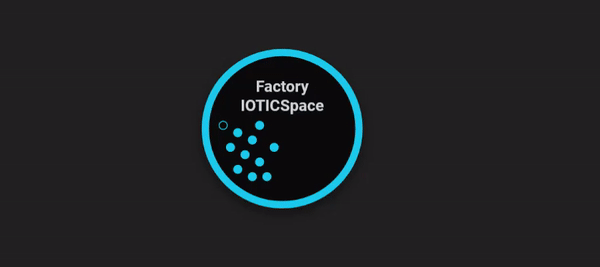## Prerequisites

Before starting you need:

## IOTICSpace

An IOTICSpace is an ecosystem of Digital Twins that enable the creation and processing of data interactions, leading into powerful, useable event analytics. With an IOTICSpace data becomes Findable, Accessible, Interoperable, and Reusable.

For the purposes of this guide all you need to know about IOTICSpaces is that they allow you to create Digital Twins quickly and consistently as well as enabling them to connect and communicate with other Digital Twins. If you would like to read more about the benefits of IOTICSpaces then take a look at our FAIR Data page for more information.

We will also be referencing a number of endpoints for your space, if you are ever unsure where to go you can find all the relevant endpoints for your IOTICSpace by going to: https://yourspace.iotics.space/index.json.

## Environment set up

To make this guide as easy to follow as possible we will be setting up a new Python Virtual Environment containing the IOTICS Host Library and all dependencies.

1. Clone the IOTICS Host Library to your device

2. In your command line navigate to the directory you cloned the library into(for example: `C:\path\iotics-host-lib`) and run:

``````python3.8 -m venv env
source env/bin/activate
pip install -U pip setuptools
pip install iotics-identity
pip install -f deps/ iotics.host.lib.sources
``````
``````py -m venv env
.\env\Scripts\activate
pip install -U pip setuptools
pip install iotics-identity
pip install -f deps/ iotics.host.lib.sources
``````

### 🚧Note:

If you setup the environment in a folder other than your cloned IOTICS Host Lib folder you may experience issues with the rest of the tutorial.

## Identity

Now that we've created the environment we'll be using in the tutorial we need to set up your credentials for securely using it.

At IOTICS we take identification and security seriously. All interactions must be identified through the use of Decentralised IDs (DIDs).

We will only be touching lightly on DIDs for this tutorial, but they are an emerging effort for establishing a standard for self-sovereign digital identities from the W3C Credentials Community Group. You can read more about DIDs on our Decentralized Identity Documents Key Concept page if you'd like to know more.

For this tutorial we will use the IOTICS Host Library to run through the process of Identification to get you up and running as quickly as possible.

1. Note the URL of the `Resolver` found in the `index.json` file of your IOTICSpace, for example: https://yourspace.iotics.space/index.json

2. Run this in the root of your Host Library directory:

``````./scripts/gen_creds.py --resolver [resolver url]
``````
1. Confirm `y` when asked: `Creating a new agent, continue? [y/n]`.

2. Take note of the output which will look something like this:

``````export RESOLVER_HOST=https://your.resolver
export USER_KEY_NAME=00
export AGENT_SEED=00010203example708090a0b0c0dexample11213141516171example1c1d1e1f
export AGENT_KEY_NAME=00
``````

#### Creating and authenticating `tutorial.py`

The first step is to create the program's `main()` method and passing our authentication credentials into an api_factory, which we'll use to interact with the IOTICS API.

For this step we'll need a number of different variables, they will come from either the script you just ran or found in the `index.json` file of your IOTICSpace(for example: https://yourspace.iotics.space/index.json).

Variable

Source

`resolver_url`

Index.json

`user_seed`

Script output

`user_key_name`

Script output

`agent_seed`

Script output

`agent_key_name`

Script output

`qapi_url`

Index.json

`qapi_stomp_url`

Index.json

Now you have all the information you need to hand we start the tutorial file:

1. Create a new filed called `tutorial.py`

2. Copy and paste the below code into this file, filling in the variables with the arguments you generated in the last step:

``````import base64
import json
from time import sleep

import requests
from iotic.web.rest.client.qapi import (
GeoLocation,
LangLiteral,
ModelProperty,
StringLiteral,
UpsertFeedWithMeta,
Uri,
Value,
Visibility,
)
from iotics.host.api.data_types import BasicDataTypes
from iotics.host.api.qapi import QApiFactory
from iotics.host.auth import AgentAuthBuilder
from iotics.host.conf.base import DataSourcesConfBase

RESOLVER_URL = "resolver_url"
QAPI_URL = "qapi_url"
QAPI_STOMP_URL = "qapi_stomp_url"

USER_KEY_NAME = "user_key_name"
AGENT_KEY_NAME = "agent_key_name"
USER_SEED = "user_seed"
AGENT_SEED = "agent_seed"

class Tutorial:
def __init__(self):
self._agent_auth = None
self._twin_api = None
self._search_api = None
self._feed_api = None
self._follow_api = None

def setup(self):
self._agent_auth = AgentAuthBuilder.build_agent_auth(
resolver_url=RESOLVER_URL,
user_seed=USER_SEED,
user_key_name=USER_KEY_NAME,
agent_seed=AGENT_SEED,
agent_key_name=AGENT_KEY_NAME,
)

api_factory = QApiFactory(
DataSourcesConfBase(qapi_url=QAPI_URL, qapi_stomp_url=QAPI_STOMP_URL),
self._agent_auth,
)
self._twin_api = api_factory.get_twin_api()
self._search_api = api_factory.get_search_api()
self._feed_api = api_factory.get_feed_api()
self._follow_api = api_factory.get_follow_api()

def main():
tutorial = Tutorial()
tutorial.setup()

if __name__ == "__main__":
main()
``````

Now that we've got our credentials in place we can move on to creating our first Digital Twin, the first step is to create Twin Model.

### Creating your first Twin Model

For this section we're going to first create a Twin Model that we can then use to quickly create a number of Twins. This allows our Factory owner to quickly model their machinery for monitoring.

#### The `create_model` method

First we're going to create a new method called `create_model` that uses the `agent_auth` and `api_factory` you previously created. To create the Model Twin and its Feed, we will be using the Twin API Service and Feed API Service.

As we want to be able to find this Twin in searches we should add some descriptions to it.

In the code snippet below we will give the Twin:

• a label
• set it's visibility to public
• add the RDF "type" property to say it's a model (a concept that exists in the IOTICS ontology)
1. Add this new method to your `tutorial` class:
``````def create_model(self):
# Create Model Twin
model_twin_id = self._agent_auth.make_twin_id("MachineModel")

# Update Twin with Metadata, Feed and Value
self._twin_api.upsert_twin(
twin_id=model_twin_id,
visibility=TWIN_VISIBILITY,
properties=[
MODEL_TYPE_PROPERTY,
ALLOW_ALL_HOSTS_PROPERTY,
ModelProperty(
key=LABEL_PREDICATE,
lang_literal_value=LangLiteral(value=MODEL_LABEL, lang="en"),
),
],
feeds=[
UpsertFeedWithMeta(
id=SOURCE_FEED_NAME,
store_last=True,
properties=[
ModelProperty(
key=LABEL_PREDICATE,
lang_literal_value=LangLiteral(
value="Current temperature", lang="en"
),
)
],
values=[
Value(
label=SOURCE_VALUE_LABEL,
comment="Temperature in degrees Celsius",
unit="http://purl.obolibrary.org/obo/UO_0000027",
data_type=BasicDataTypes.DECIMAL.value,
)
],
)
],
)

print("Model twin created")

return model_twin_id
``````

### 🚧Note:

The seed string should be unique to your space, if you reuse a seed string then the same ID will be created. This results in the API finding a pre-existing Twin when you try to create a new Twin.

We will give it a second type in your ontology to say that this is the model of your Machine class.

1. Add the below at the top of `tutorial.py`:
``````# Top of file
MODEL_TYPE_PROPERTY = ModelProperty(
key="http://www.w3.org/1999/02/22-rdf-syntax-ns#type",
uri_value=Uri(value="https://data.iotics.com/app#Model"),
)
ALLOW_ALL_HOSTS_PROPERTY = ModelProperty(
key="http://data.iotics.com/public#hostAllowList",
uri_value=Uri(value="http://data.iotics.com/public#allHosts"),
)
LABEL_PREDICATE = "http://www.w3.org/2000/01/rdf-schema#label"

MODEL_LABEL = "Machine model (tutorial)"
SOURCE_FEED_NAME = "currentTemp"
SOURCE_VALUE_LABEL = "temperature"

TWIN_VISIBILITY = Visibility.PRIVATE
``````
1. Add the `create_model` method to `main()`:
``````# continue method
def main():
tutorial = Tutorial()
tutorial.setup()

model_twin_id = tutorial.create_model()
``````
1. Now just run the tutorial file from your CLI:
``````python tutorial.py
``````

You should see something similar to the below returned: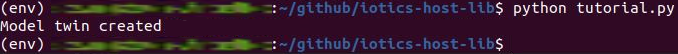### 👍Congratulations!

You have created your first model!

### Creating your first Digital Twins and connecting data

Now that we've created a Model we can use it to create the Twins needed to monitor our Factory's assets. We'll be using the same APIs as before, so this method will need the same parameters as the method used to make the model.

We'll also be using the Search API Reference, created from the api_factory the same way the other APIs are. We'll only be lightly touching on searching in this section, but if you want a more in-depth look see our dedicated Searching Guide.

1. Add this new `create_machine_from_model` method to the `tutorial` class:
``````def create_machine_from_model(self, data):
# Search for Machine Model
twins_list = self._search_api.search_twins(
properties=[MODEL_TYPE_PROPERTY], text=MODEL_LABEL
)

model_twin = next(twins_list).twins
model_twin_id = model_twin.id.value

# Create new twins based on the model
for machine_number, sensor_data in enumerate(data):
machine_name = f"machine_{machine_number}"
machine_twin_id = self._agent_auth.make_twin_id(machine_name)

# Create Twin
self._twin_api.create_twin(machine_twin_id)

# Get the Model Twin's feeds list
model_feeds_list = model_twin.feeds
# Get the id of the first (and only) feed in the list
feed_id = next(iter(model_feeds_list)).feed.id.value
# Describe the feed to get metadata and properties
feed_info = self._feed_api.describe_feed(
twin_id=model_twin_id, feed_id=feed_id
).result

# Update Twin with Metadata, Feed(s) and Value(s)
self._twin_api.upsert_twin(
twin_id=machine_twin_id,
visibility=TWIN_VISIBILITY,
location=GeoLocation(lat=51.5, lon=-0.1),
properties=[
ALLOW_ALL_HOSTS_PROPERTY,
ModelProperty(
key=LABEL_PREDICATE,
lang_literal_value=LangLiteral(
value=f"{machine_name} (tutorial)", lang="en"
),
),
ModelProperty(
key="http://www.w3.org/1999/02/22-rdf-syntax-ns#type",
uri_value=Uri(value="https://data.iotics.com/tutorial#Sensor"),
),
ModelProperty(
key="https://data.iotics.com/app#model",
uri_value=Uri(value=str(model_twin_id)),
),
ModelProperty(
key="https://data.iotics.com/tutorial#serialNumber",
string_literal_value=StringLiteral(
value="%06d" % machine_number
),
),
],
feeds=[
UpsertFeedWithMeta(
id=feed_id,
store_last=feed_info.store_last,
properties=feed_info.properties,
values=feed_info.values,
)
],
)

# Share first sample of data
self._publish_feed_value(
sensor_data, twin_id=machine_twin_id, feed_id=feed_id, print_data=False
)

SENSORS_MAP[machine_name] = machine_twin_id

print("Machine twin created:", machine_name)

def _publish_feed_value(
self, sensor_data, twin_id, feed_id, print_data=True, twin_label=None
):
data_to_share = {SOURCE_VALUE_LABEL: sensor_data["temp"]}
encoded_data = base64.b64encode(json.dumps(data_to_share).encode()).decode()

self._feed_api.share_feed_data(
twin_id, feed_id, data=encoded_data, mime="application/json"
)

if print_data:
print(f"Sharing data for {twin_label}: {data_to_share}")
``````

For this tutorial we have provided a mock data source for our scenario: an API which gives temperature readings for ten sensors. To bring the tutorial scenario to life two of these sensors(number 6 and number 10) will gradually overheat.

1. Add the new `get_sensor_data` method the `tutorial` class:
``````def get_sensor_data(self):
if response.status_code > 400:
print(f"Error {response.status_code} from API: {response.reason}")

return response.json()
``````
``````# Top of file
SUBSCRIPTIONS_MAP = {}
SENSORS_MAP = {}
``````

Now we can find our Model so its structure can determine the structure of Twins in the class it defines.

1. Add this to the bottom of `main()`:
``````def main():
tutorial = Tutorial()
tutorial.setup()

model_twin_id = tutorial.create_model()
data = tutorial.get_sensor_data()
tutorial.create_machine_from_model(data)
``````
1. Now run the script to make these changes in your space:
``````python tutorial.py
``````

You should see something similar to the below returned: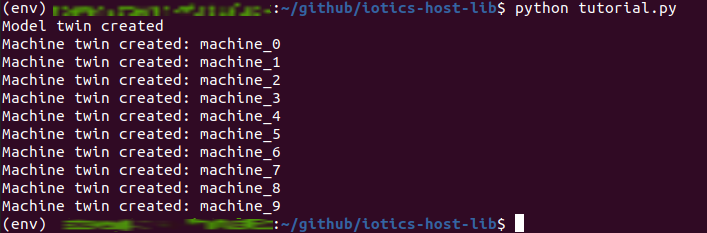### Sharing data

For each sensor in the API output, it should ensure the Twin is in place, with the appropriate name. To do so it needs to parse the data to match the Values we used when defining the feed: a single numeric value, keyed by 'temperature'.

1. Create the `share_data` method to the `tutorial` class:
``````def share_data(self, data):
for machine_number, sensor_data in enumerate(data):
machine_name = f"machine_{machine_number}"
machine_twin_id = SENSORS_MAP.get(machine_name)
if not machine_twin_id:
continue

self._publish_feed_value(
sensor_data,
twin_id=machine_twin_id,
feed_id=SOURCE_FEED_NAME,
twin_label=machine_name,
)
``````
1. Add this loop to the bottom of `main()`:
``````def main():
tutorial = Tutorial()
tutorial.setup()

model_twin_id = tutorial.create_model()
data = tutorial.get_sensor_data()
tutorial.create_machine_from_model(data)
interaction_twin_id = tutorial.create_interaction(model_twin_id)
tutorial.follow_sensors(interaction_twin_id)

while True:
print("\nGetting latest temperatures...")
data = tutorial.get_sensor_data()
tutorial.share_data(data)

sleep(5)
``````
1. Run the script:
``````python tutorial.py
``````

You'll see the script repeatedly call the API, creating any twins not already in place, and sharing the relevant data: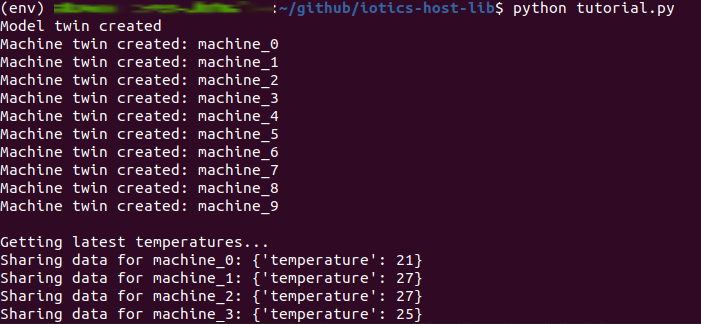## Creating interactions

Data interactions allow you to combine or transform data in various ways to generate new data that is more specific to your needs. In this example, we are going to do a Transformation Interaction on the machine Twins we created earlier, then create new Twins which alert us if the temperature gets too hot.

We do not need to create these output twins ourselves as there is a process running in your IOTICSpace which automatically does this for you. All you need to do is create an Interaction Twin with the appropriate configuration.

As the last 3 must match values we are using elsewhere, we will need to extract them to global variables.

1. Add the below at the top of `tutorial.py`:
``````# Top of file
OUTPUT_FEED_NAME = "temperature_status"
OUTPUT_VALUE_LABEL = "status"
``````
1. Add the new `create_interaction` method to the `tutorial` class:
``````def create_interaction(self, model_twin_id):
# Create Interaction Twin
interaction_twin_id = self._agent_auth.make_twin_id("SensorInteraction")

# Setup Interaction config
interaction_config = {
"enabled": True,
"rules": [
{
"transformation": {
"conditions": [
{
"fieldsIncludedInOutput": [SOURCE_VALUE_LABEL],
"jsonLogic": {">": [{"var": SOURCE_VALUE_LABEL}, 30]},
}
],
"outputFeedId": OUTPUT_FEED_NAME,
"outputFieldId": OUTPUT_VALUE_LABEL,
"outputTrueValue": "extreme",
"outputFalseValue": "normal",
"sourceFeedId": SOURCE_FEED_NAME,
"sourceId": "1",
}
}
],
"sources": [
{
"cleanupRateS": 900,
"feeds": [
{"fieldIds": [SOURCE_VALUE_LABEL], "id": SOURCE_FEED_NAME}
],
"filter": {
"properties": [
{
"key": "https://data.iotics.com/app#model",
"value": {"uriValue": {"value": model_twin_id}},
}
],
"text": None,
},
"id": "1",
"modelDid": model_twin_id,
"refreshRateS": 300,
}
],
}

# Update Twin with Metadata, Feed and Value
self._twin_api.upsert_twin(
twin_id=interaction_twin_id,
visibility=TWIN_VISIBILITY,
properties=[
MODEL_TYPE_PROPERTY,
ALLOW_ALL_HOSTS_PROPERTY,
ModelProperty(
key="http://www.w3.org/1999/02/22-rdf-syntax-ns#type",
uri_value=Uri(value="https://data.iotics.com/app#Interaction"),
),
ModelProperty(
key="https://data.iotics.com/app#interactionConfig",
string_literal_value=StringLiteral(
value=json.dumps(interaction_config)
),
),
ModelProperty(
key=LABEL_PREDICATE,
lang_literal_value=LangLiteral(
),
),
],
feeds=[
UpsertFeedWithMeta(
id=OUTPUT_FEED_NAME,
store_last=True,
properties=[
ModelProperty(
key=LABEL_PREDICATE,
lang_literal_value=LangLiteral(
value="Temperature status", lang="en"
),
)
],
values=[
Value(
label=OUTPUT_VALUE_LABEL,
comment="Temperature status: normal or extreme",
data_type=BasicDataTypes.STRING.value,
)
],
)
],
)

print("Interaction twin created")

return interaction_twin_id
``````

We need to populate this twin so the Interaction Engine can find it. To do this we give it the required RDF type. The above config is added in another property.

1. Add this method to `main()` before the while loop:
``````def main():
tutorial = Tutorial()
tutorial.setup()

model_twin_id = tutorial.create_model()
data = tutorial.get_sensor_data()
tutorial.create_machine_from_model(data)
interaction_twin_id = tutorial.create_interaction(model_twin_id)

while True:
print("\nGetting latest temperatures...")
data = tutorial.get_sensor_data()
tutorial.share_data(data)

sleep(5)
``````

If you run this file, you will

1. Run this file:
``````python tutorial.py
``````

You'll see the Interaction Twin created within your space: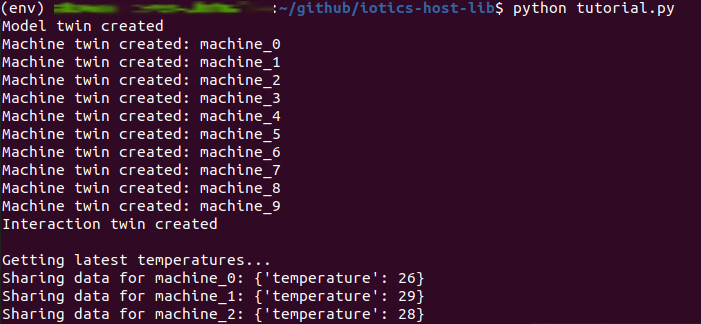### 👍Congratulations!

You have created your first Interaction Twin!

The last step in our tutorial will be to follow the new output Twins so we can be alerted whenever one of our machines overheats. In practice this would usually be be in a different process from the one reading the data into IOTICSpace, but to minimize how many tabs you have to work with, the tutorial is going to put it together in the same script.

Here we use a fourth IOTICS API, the Follow API.

1. Add the `follow_sensors` method to the `tutorial` class:`:
``````def follow_sensors(self, interaction_twin_id):
output_twins = []

print("Searching for output twins", end="", flush=True)
sleep(10)

while len(output_twins) < len(SENSORS_MAP):
output_twins = next(
self._search_api.search_twins(
properties=[
ModelProperty(
key="https://data.iotics.com/app#model",
uri_value=Uri(value=interaction_twin_id),
)
]
)
).twins

sleep(10)
print(".", end="", flush=True)

print(f"\nFound {len(output_twins)} output twins")

for sensor in output_twins:
subscription_id = self._follow_api.subscribe_to_feed(
follower_twin_id=sensor.id.value,
followed_twin_id=sensor.id.value,
followed_feed_name=OUTPUT_FEED_NAME,
callback=self._follow_callback,
)
sensor_label = self._find_label(properties=sensor.properties)

if sensor_label:
SUBSCRIPTIONS_MAP[subscription_id] = sensor_label

def _find_label(self, properties):
for prop in properties:
if prop.key == LABEL_PREDICATE:
return prop.lang_literal_value.value

return None
``````

We can find all the output Twins by searching for the property which specifies a twin's model.

Now we're going to use that follow_api to provide a callback method that gets called whenever data is shared to the specified feed. The callback will need to know which Twin is triggering it, so we save the "subscription ids" we get from following Twins into a dict with the Twins' labels, which we make globally available.

For the tutorial we'll add a simple sample logic for the callback method to print a message to the console whenever a sensor overheats.

You could do any number of things instead, send an email, make a POST request to an API managing the sensors, even share data to another twin instead.

1. Add the new `follow_callback` method:
``````def _follow_callback(self, sub_id, body):
sensor = SUBSCRIPTIONS_MAP[sub_id]
)

if interaction_data[OUTPUT_VALUE_LABEL] == "extreme":
print(f"{sensor}: SENSOR IS OVERHEATING! OH THE HUMANITY!!")
``````
1. Add the new `follow_sensors` method just before the infinite loop in `main()`:
``````):
tutorial = Tutorial()
tutorial.setup()

model_twin_id = tutorial.create_model()
data = tutorial.get_sensor_data()
tutorial.create_machine_from_model(data)
interaction_twin_id = tutorial.create_interaction(model_twin_id)
tutorial.follow_sensors(interaction_twin_id)

while True:
print("\nGetting latest temperatures...")
data = tutorial.get_sensor_data()
tutorial.share_data(data)

sleep(5)
``````
1. Now run the script:
``````python tutorial.py
``````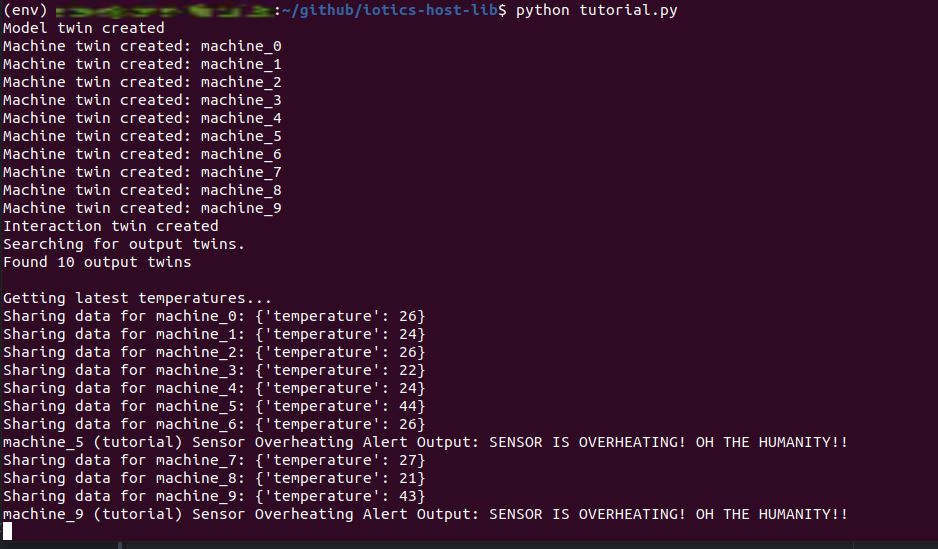### 👍Congratulations!

Your data interaction is now running end-to-end, and the tutorial is complete!

## Example file

Click to see example tutorial.py

``````import base64
import json
from time import sleep

import requests
from iotic.web.rest.client.qapi import (
GeoLocation,
LangLiteral,
ModelProperty,
StringLiteral,
UpsertFeedWithMeta,
Uri,
Value,
Visibility,
)
from iotics.host.api.data_types import BasicDataTypes
from iotics.host.api.qapi import QApiFactory
from iotics.host.auth import AgentAuthBuilder
from iotics.host.conf.base import DataSourcesConfBase

RESOLVER_URL = "resolver_url"
QAPI_URL = "qapi_url"
QAPI_STOMP_URL = "qapi_stomp_url"

USER_KEY_NAME = "user_key_name"
AGENT_KEY_NAME = "agent_key_name"
USER_SEED = "user_seed"
AGENT_SEED = "agent_seed"

MODEL_TYPE_PROPERTY = ModelProperty(
key="http://www.w3.org/1999/02/22-rdf-syntax-ns#type",
uri_value=Uri(value="https://data.iotics.com/app#Model"),
)
ALLOW_ALL_HOSTS_PROPERTY = ModelProperty(
key="http://data.iotics.com/public#hostAllowList",
uri_value=Uri(value="http://data.iotics.com/public#allHosts"),
)
LABEL_PREDICATE = "http://www.w3.org/2000/01/rdf-schema#label"

MODEL_LABEL = "Machine model (tutorial)"
SOURCE_FEED_NAME = "currentTemp"
SOURCE_VALUE_LABEL = "temperature"
OUTPUT_FEED_NAME = "temperature_status"
OUTPUT_VALUE_LABEL = "status"
SUBSCRIPTIONS_MAP = {}
SENSORS_MAP = {}

TWIN_VISIBILITY = Visibility.PRIVATE

class Tutorial:
def __init__(self):
self._agent_auth = None
self._twin_api = None
self._search_api = None
self._feed_api = None
self._follow_api = None

def setup(self):
self._agent_auth = AgentAuthBuilder.build_agent_auth(
resolver_url=RESOLVER_URL,
user_seed=USER_SEED,
user_key_name=USER_KEY_NAME,
agent_seed=AGENT_SEED,
agent_key_name=AGENT_KEY_NAME,
)

api_factory = QApiFactory(
DataSourcesConfBase(qapi_url=QAPI_URL, qapi_stomp_url=QAPI_STOMP_URL),
self._agent_auth,
)
self._twin_api = api_factory.get_twin_api()
self._search_api = api_factory.get_search_api()
self._feed_api = api_factory.get_feed_api()
self._follow_api = api_factory.get_follow_api()

def create_model(self):
# Create Model Twin
model_twin_id = self._agent_auth.make_twin_id("MachineModel")

# Update Twin with Metadata, Feed and Value
self._twin_api.upsert_twin(
twin_id=model_twin_id,
visibility=TWIN_VISIBILITY,
properties=[
MODEL_TYPE_PROPERTY,
ALLOW_ALL_HOSTS_PROPERTY,
ModelProperty(
key=LABEL_PREDICATE,
lang_literal_value=LangLiteral(value=MODEL_LABEL, lang="en"),
),
],
feeds=[
UpsertFeedWithMeta(
id=SOURCE_FEED_NAME,
store_last=True,
properties=[
ModelProperty(
key=LABEL_PREDICATE,
lang_literal_value=LangLiteral(
value="Current temperature", lang="en"
),
)
],
values=[
Value(
label=SOURCE_VALUE_LABEL,
comment="Temperature in degrees Celsius",
unit="http://purl.obolibrary.org/obo/UO_0000027",
data_type=BasicDataTypes.DECIMAL.value,
)
],
)
],
)

print("Model twin created")

return model_twin_id

def create_machine_from_model(self, data):
# Search for Machine Model
twins_list = self._search_api.search_twins(
properties=[MODEL_TYPE_PROPERTY], text=MODEL_LABEL
)

model_twin = next(twins_list).twins
model_twin_id = model_twin.id.value

# Create new twins based on the model
for machine_number, sensor_data in enumerate(data):
machine_name = f"machine_{machine_number}"
machine_twin_id = self._agent_auth.make_twin_id(machine_name)

# Create Twin
self._twin_api.create_twin(machine_twin_id)

# Get the Model Twin's feeds list
model_feeds_list = model_twin.feeds
# Get the id of the first (and only) feed in the list
feed_id = next(iter(model_feeds_list)).feed.id.value
# Describe the feed to get metadata and properties
feed_info = self._feed_api.describe_feed(
twin_id=model_twin_id, feed_id=feed_id
).result

# Update Twin with Metadata, Feed(s) and Value(s)
self._twin_api.upsert_twin(
twin_id=machine_twin_id,
visibility=TWIN_VISIBILITY,
location=GeoLocation(lat=51.5, lon=-0.1),
properties=[
ALLOW_ALL_HOSTS_PROPERTY,
ModelProperty(
key=LABEL_PREDICATE,
lang_literal_value=LangLiteral(
value=f"{machine_name} (tutorial)", lang="en"
),
),
ModelProperty(
key="http://www.w3.org/1999/02/22-rdf-syntax-ns#type",
uri_value=Uri(value="https://data.iotics.com/tutorial#Sensor"),
),
ModelProperty(
key="https://data.iotics.com/app#model",
uri_value=Uri(value=str(model_twin_id)),
),
ModelProperty(
key="https://data.iotics.com/tutorial#serialNumber",
string_literal_value=StringLiteral(
value="%06d" % machine_number
),
),
],
feeds=[
UpsertFeedWithMeta(
id=feed_id,
store_last=feed_info.store_last,
properties=feed_info.properties,
values=feed_info.values,
)
],
)

# Share first sample of data
self._publish_feed_value(
sensor_data, twin_id=machine_twin_id, feed_id=feed_id, print_data=False
)

SENSORS_MAP[machine_name] = machine_twin_id

print("Machine twin created:", machine_name)

def create_interaction(self, model_twin_id):
# Create Interaction Twin
interaction_twin_id = self._agent_auth.make_twin_id("SensorInteraction")

# Setup Interaction config
interaction_config = {
"enabled": True,
"rules": [
{
"transformation": {
"conditions": [
{
"fieldsIncludedInOutput": [SOURCE_VALUE_LABEL],
"jsonLogic": {">": [{"var": SOURCE_VALUE_LABEL}, 30]},
}
],
"outputFeedId": OUTPUT_FEED_NAME,
"outputFieldId": OUTPUT_VALUE_LABEL,
"outputTrueValue": "extreme",
"outputFalseValue": "normal",
"sourceFeedId": SOURCE_FEED_NAME,
"sourceId": "1",
}
}
],
"sources": [
{
"cleanupRateS": 900,
"feeds": [
{"fieldIds": [SOURCE_VALUE_LABEL], "id": SOURCE_FEED_NAME}
],
"filter": {
"properties": [
{
"key": "https://data.iotics.com/app#model",
"value": {"uriValue": {"value": model_twin_id}},
}
],
"text": None,
},
"id": "1",
"modelDid": model_twin_id,
"refreshRateS": 300,
}
],
}

# Update Twin with Metadata, Feed and Value
self._twin_api.upsert_twin(
twin_id=interaction_twin_id,
visibility=TWIN_VISIBILITY,
properties=[
MODEL_TYPE_PROPERTY,
ALLOW_ALL_HOSTS_PROPERTY,
ModelProperty(
key="http://www.w3.org/1999/02/22-rdf-syntax-ns#type",
uri_value=Uri(value="https://data.iotics.com/app#Interaction"),
),
ModelProperty(
key="https://data.iotics.com/app#interactionConfig",
string_literal_value=StringLiteral(
value=json.dumps(interaction_config)
),
),
ModelProperty(
key=LABEL_PREDICATE,
lang_literal_value=LangLiteral(
),
),
],
feeds=[
UpsertFeedWithMeta(
id=OUTPUT_FEED_NAME,
store_last=True,
properties=[
ModelProperty(
key=LABEL_PREDICATE,
lang_literal_value=LangLiteral(
value="Temperature status", lang="en"
),
)
],
values=[
Value(
label=OUTPUT_VALUE_LABEL,
comment="Temperature status: normal or extreme",
data_type=BasicDataTypes.STRING.value,
)
],
)
],
)

print("Interaction twin created")

return interaction_twin_id

def follow_sensors(self, interaction_twin_id):
output_twins = []

print("Searching for output twins", end="", flush=True)
sleep(10)

while len(output_twins) < len(SENSORS_MAP):
output_twins = next(
self._search_api.search_twins(
properties=[
ModelProperty(
key="https://data.iotics.com/app#model",
uri_value=Uri(value=interaction_twin_id),
)
]
)
).twins

sleep(10)
print(".", end="", flush=True)

print(f"\nFound {len(output_twins)} output twins")

for sensor in output_twins:
subscription_id = self._follow_api.subscribe_to_feed(
follower_twin_id=sensor.id.value,
followed_twin_id=sensor.id.value,
followed_feed_name=OUTPUT_FEED_NAME,
callback=self._follow_callback,
)
sensor_label = self._find_label(properties=sensor.properties)

if sensor_label:
SUBSCRIPTIONS_MAP[subscription_id] = sensor_label

def get_sensor_data(self):
if response.status_code > 400:
print(f"Error {response.status_code} from API: {response.reason}")

return response.json()

def share_data(self, data):
for machine_number, sensor_data in enumerate(data):
machine_name = f"machine_{machine_number}"
machine_twin_id = SENSORS_MAP.get(machine_name)
if not machine_twin_id:
continue

self._publish_feed_value(
sensor_data,
twin_id=machine_twin_id,
feed_id=SOURCE_FEED_NAME,
twin_label=machine_name,
)

def _publish_feed_value(
self, sensor_data, twin_id, feed_id, print_data=True, twin_label=None
):
data_to_share = {SOURCE_VALUE_LABEL: sensor_data["temp"]}
encoded_data = base64.b64encode(json.dumps(data_to_share).encode()).decode()

self._feed_api.share_feed_data(
twin_id, feed_id, data=encoded_data, mime="application/json"
)

if print_data:
print(f"Sharing data for {twin_label}: {data_to_share}")

def _follow_callback(self, sub_id, body):
sensor = SUBSCRIPTIONS_MAP[sub_id]
)

if interaction_data[OUTPUT_VALUE_LABEL] == "extreme":
print(f"{sensor}: SENSOR IS OVERHEATING! OH THE HUMANITY!!")

def _find_label(self, properties):
for prop in properties:
if prop.key == LABEL_PREDICATE:
return prop.lang_literal_value.value

return None

def main():
tutorial = Tutorial()
tutorial.setup()

model_twin_id = tutorial.create_model()
data = tutorial.get_sensor_data()
tutorial.create_machine_from_model(data)
interaction_twin_id = tutorial.create_interaction(model_twin_id)
tutorial.follow_sensors(interaction_twin_id)

while True:
print("\nGetting latest temperatures...")
data = tutorial.get_sensor_data()
tutorial.share_data(data)

sleep(5)

if __name__ == "__main__":
main()
``````